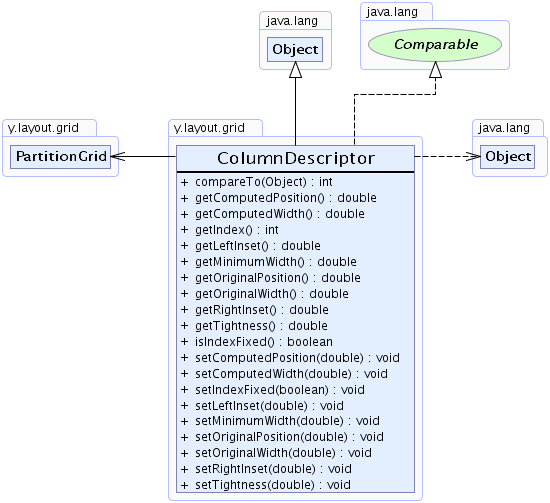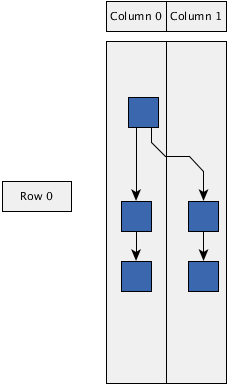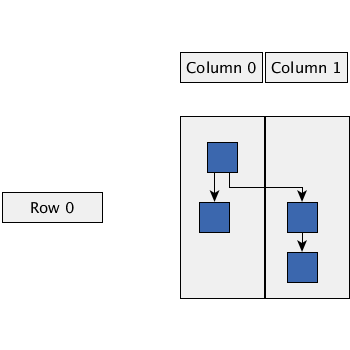Search this API

## y.layout.grid Class ColumnDescriptor

```java.lang.Objecty.layout.grid.ColumnDescriptor
```
All Implemented Interfaces:
java.lang.Comparable

`public class ColumnDescriptorextends java.lang.Objectimplements java.lang.Comparable`

This class represents a column of a `partition grid` structure.

The results of the calculation of the geometry of the column will be placed into the instances of this class after the layout.

`PartitionGrid`Method Summary
` int` `compareTo(java.lang.Object o)`
Compares this `ColumnDescriptor` instance with a given other `ColumnDescriptor` instance based on the indices of the two instances.
` double` `getComputedPosition()`
Returns the computed position (smallest x-coordinate) of the column after the layout has been calculated.
` double` `getComputedWidth()`
Returns the computed width of the column after the layout has been calculated.
` int` `getIndex()`
Returns the index of the column within the `partition grid`.
` double` `getLeftInset()`
Returns the left column inset in which no element will lie in the resulting layout.
` double` `getMinimumWidth()`
Returns the minimum width of the column.
` double` `getOriginalPosition()`
Returns the original position (smallest x-coordinate) of the column.
` double` `getOriginalWidth()`
Returns the original width of the column.
` double` `getRightInset()`
Returns the right column inset in which no element will lie in the resulting layout.
` double` `getTightness()`
Returns the tightness factor of this column.
` boolean` `isIndexFixed()`
Returns whether the index of the column is fixed or it should be chosen automatically in order to minimize edge lengths.
` void` `setComputedPosition(double computedPosition)`
Specifies the computed position (smallest x-coordinate) of the column after the layout has been calculated.
` void` `setComputedWidth(double computedWidth)`
Specifies the computed width of the column after the layout has been calculated.
` void` `setIndexFixed(boolean indexFixed)`
Specifies whether the index of the column is fixed or it should be chosen automatically in order to minimize edge lengths.
` void` `setLeftInset(double leftInset)`
Specifies the left column inset in which no element will lie in the resulting layout.
` void` `setMinimumWidth(double minimumWidth)`
Specifies the minimum width of the column.
` void` `setOriginalPosition(double originalPosition)`
Specifies the original position (smallest x-coordinate) of the column.
` void` `setOriginalWidth(double originalWidth)`
Specifies the original width of the column.
` void` `setRightInset(double rightInset)`
Specifies the right column inset in which no element will lie in the resulting layout.
` void` `setTightness(double tightness)`
Specifies the tightness factor of this column.

Methods inherited from class java.lang.Object
`clone, equals, finalize, getClass, hashCode, notify, notifyAll, toString, wait, wait, wait`

Method Detail

### getIndex

`public int getIndex()`
Returns the index of the column within the `partition grid`.

Returns:
the index of the column

### compareTo

`public int compareTo(java.lang.Object o)`
Compares this `ColumnDescriptor` instance with a given other `ColumnDescriptor` instance based on the indices of the two instances.

Specified by:
`compareTo` in interface `java.lang.Comparable`
Parameters:
`o` - the `ColumnDescriptor` to compare to
Returns:
`-1`, `0` or `1` if this `ColumnDescriptor` is less than, equal to, or greater than the given other `ColumnDescriptor`

### getMinimumWidth

`public double getMinimumWidth()`
Returns the minimum width of the column.

The minimum width is defined to be at least `0`.

Returns:
the minimum column width
`setMinimumWidth(double)`

### setMinimumWidth

`public void setMinimumWidth(double minimumWidth)`
Specifies the minimum width of the column.

The minimum width is defined to be at least `0`.

Default Value:
The default value is 0.0.
Parameters:
`minimumWidth` - the minimum column width
Throws:
`java.lang.IllegalArgumentException` - if the minimum width is negative
Sample Graphs:Minimum column width `0`Minimum column width `100`

### getLeftInset

`public double getLeftInset()`
Returns the left column inset in which no element will lie in the resulting layout.

The column inset must be greater than or equal to `0`.

Returns:
the left column inset
`setLeftInset(double)`

### setLeftInset

`public void setLeftInset(double leftInset)`
Specifies the left column inset in which no element will lie in the resulting layout.

The column inset must be greater than or equal to `0`.

Default Value:
The default value is 0.0.
Parameters:
`leftInset` - the left column inset
Throws:
`java.lang.IllegalArgumentException` - if the left inset is negative
Sample Graphs:All insets `10`All insets `50`

### getRightInset

`public double getRightInset()`
Returns the right column inset in which no element will lie in the resulting layout.

The column inset must be greater than or equal to `0`.

Returns:
the right column inset
`setRightInset(double)`

### setRightInset

`public void setRightInset(double rightInset)`
Specifies the right column inset in which no element will lie in the resulting layout.

The column inset must be greater than or equal to `0`.

Default Value:
The default value is 0.0.
Parameters:
`rightInset` - the right column inset
Throws:
`java.lang.IllegalArgumentException` - if the right inset is negative
Sample Graphs:All insets `10`All insets `50`

### getComputedWidth

`public double getComputedWidth()`
Returns the computed width of the column after the layout has been calculated.

The computed width needs to be greater than or equal to `0`.

Returns:
the computed width
`setComputedWidth(double)`

### setComputedWidth

`public void setComputedWidth(double computedWidth)`
Specifies the computed width of the column after the layout has been calculated.

The computed width needs to be greater than or equal to `0`.

Default Value:
The default value is 0.0.
Parameters:
`computedWidth` - the computed width
Throws:
`java.lang.IllegalArgumentException` - if the computed width is negative

### getOriginalWidth

`public double getOriginalWidth()`
Returns the original width of the column.

The original width must be greater than or equal to `0`.

Returns:
the original width
`setOriginalWidth(double)`

### setOriginalWidth

`public void setOriginalWidth(double originalWidth)`
Specifies the original width of the column.

The original width should be greater than or equal to `0`.

Default Value:
The default value is 0.0.
Parameters:
`originalWidth` - the original width
Throws:
`java.lang.IllegalArgumentException` - if the original width is negative

### getOriginalPosition

`public double getOriginalPosition()`
Returns the original position (smallest x-coordinate) of the column.

Returns:
the original position (smallest x-coordinate)
`setOriginalPosition(double)`

### setOriginalPosition

`public void setOriginalPosition(double originalPosition)`
Specifies the original position (smallest x-coordinate) of the column.

Default Value:
The default value is 0.0.
Parameters:
`originalPosition` - the original position (smallest x-coordinate)

### getComputedPosition

`public double getComputedPosition()`
Returns the computed position (smallest x-coordinate) of the column after the layout has been calculated.

Returns:
the computed position (smallest x-coordinate)
`setComputedPosition(double)`

### setComputedPosition

`public void setComputedPosition(double computedPosition)`
Specifies the computed position (smallest x-coordinate) of the column after the layout has been calculated.

Default Value:
The default value is 0.0.
Parameters:
`computedPosition` - the computed position (smallest x-coordinate)

### getTightness

`public double getTightness()`
Returns the tightness factor of this column.

This value must lie within the interval `[0,1]`.

The larger the value, the more the column will be forced to obtain its minimum width:

• a value of `0.0d` will disable the compression of the column.
• a value of `1.0d` will try to force the column to obtain its specified `minimum width`.

Returns:
a tightness value from the interval `[0,1]`
`setTightness(double)`

### setTightness

`public void setTightness(double tightness)`
Specifies the tightness factor of this column.

This value must lie within the interval `[0,1]`.

The larger the value, the more the column will be forced to obtain its minimum width:

• a value of `0.0d` will disable the compression of the column.
• a value of `1.0d` will try to force the column to obtain its specified `minimum width`.

Default Value:
The default value is 0.0. The column will not be compressed.
Parameters:
`tightness` - a tightness value from the interval `[0,1]`
Throws:
`java.lang.IllegalArgumentException` - if the tightness value is outside the interval `[0,1]`

### isIndexFixed

`public boolean isIndexFixed()`
Returns whether the index of the column is fixed or it should be chosen automatically in order to minimize edge lengths.

For all columns where this property is enabled, the relative ordering given by the indices is preserved. The remaining columns may be sorted again so that the overall edge lengths are minimized.

Returns:
`true` if the column index should be fixed, `false` if it may be chosen automatically
`setIndexFixed(boolean)`

### setIndexFixed

`public void setIndexFixed(boolean indexFixed)`
Specifies whether the index of the column is fixed or it should be chosen automatically in order to minimize edge lengths.

For all columns where this property is enabled, the relative ordering given by the indices is preserved. The remaining columns may be sorted again so that the overall edge lengths are minimized.

Default Value:
The default value is true. The index of the column is fixed.
Parameters:
`indexFixed` - `true` if the column index should be fixed, `false` if it may be chosen automatically